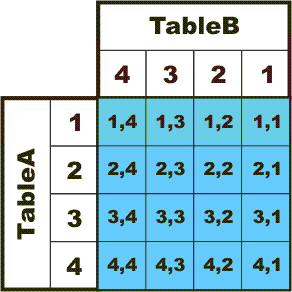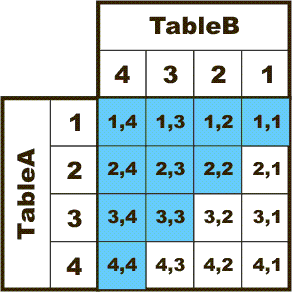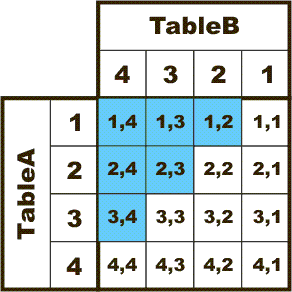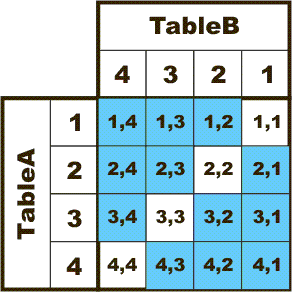# Hidden RBAR: Triangular Joins

,

## Introduction

If you've been around the forums on SQLServerCentral.com, you've probably seen me use the term "RBAR". "RBAR" is pronounced "ree-bar" and is a "Modenism" for "Row-By-Agonizing-Row". I use the term "RBAR" for anything that isn't set based. Some others have picked up on it because it's easy to remember as a form of "Death By SQL".

Many folks think that "set-based" programming means one of two things...

1. It's all done in a single query or...
2. It simply doesn't have a Cursor or a While Loop

Both of those are very bad misconceptions as to what set based programming is.

What is set based programming? Obviously, it does involve "avoiding the loop", but it's not that simple. To me, it also means that you touch each row of data only once if you can and as few times as possible if you can't. I know... sounds like a loop. And a loop will, in fact, touch a row only once... but a loop works row-by-agonizing-row, overrides the natural abilities of the optimizer, and only operates on a single row instead of a set of rows. Explicit loops are not set based programming and they just crush performance.

So, good intentioned folks have found some very clever ways of "avoiding the loop" with some pretty severe consequences. For example, they'll do something like this to do a running total and a running count...

```    USE NorthWind
SELECT x.OrderID,         x.Freight,         (SELECT SUM(y.Freight)              FROM dbo.Orders y
WHERE y.OrderID <= x.OrderID) AS RunningTotal,        (SELECT COUNT(y.Freight)
FROM dbo.Orders y
WHERE y.OrderID <= x.OrderID) AS RunningCount  FROM dbo.Orders X ORDER BY x.OrderID
```

They'll test that with a handful of rows, see that it produces the correct answer, maybe look at the Estimated Execution Plan, and walk away happy NOT realizing that they've just created a performance time bomb known as a "Triangular Join". It's not set based programming even if it looks like it. It's hidden RBAR and it can be hundreds and, sometimes, thousands of times worse than any Cursor or Loop that you'll ever write. Here's why...

## Review of Cross Joins

Before you can understand what a "Triangular Join" is, you have to become familiar with what a Cross-Join is. If you already know, you might still want to have a look at this.

Let's say you have two tables that look like this...

``` CREATE TABLE TableA (RowNum INT) INSERT INTO TableA (RowNum) SELECT 1 UNION ALL SELECT 2 UNION ALL SELECT 3 UNION ALL SELECT 4
CREATE TABLE TableB (RowNum INT) INSERT INTO TableB (RowNum) SELECT 1 UNION ALL SELECT 2 UNION ALL SELECT 3 UNION ALL SELECT 4
```

We can create an intentional Cross-Join (sometimes called a Cartesian Join) between the two tables that will return every combination of A and B (also known as a Cartesian Product) using either of the two following code snippets...

```--===== A cross-join that produces 16 combinations SELECT a.RowNum AS RowNum_A,b.RowNum AS RowNum_B   FROM TableA a,        TableB b
--===== Same cross-join using ANSI syntax produces 16 combinations SELECT a.RowNum AS RowNum_A,b.RowNum AS RowNum_B   FROM TableA a  CROSS JOIN        TableB b
```

The results of both Cross-Joins form a result set that can be depicted much as you would with a simple multiplication table (with 1 axis backwards from the other) like this... the blue area identifies all combination returns from the Cross-Join. This is why the formula for the number of internally spawned rows for a Cross-Join is simply X2 for equal length tables or A*B for unequal length tables...As you can see by the blue area... another lesser known name for a Cross-Join is a "Square Join".

## "Triangular" Joins

A Triangular Join is nothing more than about half of a Cross-Join. The "shape" of the Triangular Join depends on the relationship of < or >, <= or >=, or < > between the two tables. For very small counts, the number of internal rows spawned are nearly trivial. But, it doesn't take very many rows to spawn nightmarish numbers of internal rows that can bring a server to its knees. Here's what each type of Triangular Join can look like in code and graphically...

### <= or >= or "Equality" Triangular Join

If the Triangular Join has both an inequality and an equality in it, as in the following code example...

```--===== A triangular-join that produces 10 combinations SELECT a.RowNum AS RowNum_A,b.RowNum AS RowNum_B   FROM TableA a,        TableB b  WHERE a.RowNum <= b.RowNum
```

...the result set can be represented as in the blue area of the following graphic... notice that it forms a triangle and that's why these are called "Triangular Joins". The formula for the number of internal rows spawned for this type of Triangular Join is (X2+X)/2 (the old "sum of the sequential numbers formula")...### < or > "Inequality" Triangular Join

If your code snippet is sans an equality, like this...

```--===== A triangular-join that produces 6 combinations SELECT a.RowNum AS RowNum_A,b.RowNum AS RowNum_B   FROM TableA a,        TableB b  WHERE a.RowNum < b.RowNum
```

... you still end up with a Triangular Join, but slightly smaller. If the tables have the same number of rows, the number of internal rows generated is calculated by the formula of (X2-X)/2 and is represented by the blue area in the following graphic...### < > "Not Equal" Triangular Join - Double the Trouble!

Last, but not least, you can do the "double" triangular join using a full inequality... this is great for making schedules of teams to play teams and is represented by the following code snippet...

```--===== A triangular-join that produces 12 combinations SELECT a.RowNum AS RowNum_A,b.RowNum AS RowNum_B   FROM TableA a,        TableB b  WHERE a.RowNum <> b.RowNum
```

...and, from the following graphic, you can see why it's called a "double" triangular join. Because of the inequality, it makes good team play schedules because no team ever plays against itself... the formula to calculate rows is simply X2-X.## The Trouble with Triangles (or, the Execution Plan Lies)

Many people use triangular joins to do things like make row numbers in their code. The problem is the number of internal rows the code generates to produce the row numbers (some call this a running count).take the following code, for example...

```--===== Create a small demo table CREATE TABLE TableC (RowNum INT) INSERT INTO TableC (RowNum) SELECT 84 UNION ALL SELECT 5 UNION ALL SELECT 99 UNION ALL SELECT 12
```

... and, let's list all the values with a running count...

```--===== List the items with a running count SELECT RunCount = (SELECT COUNT(*)
FROM TableC cs
WHERE cs.SomeVal <= c.SomeVal),        c.SomeVal   FROM TableC c  ORDER BY RunCount
```

Notice the correlated subquery (a query that makes reference outside itself) and the big red triangular join it makes with the outer query? Although only 4 rows are displayed as the result set, the number of INNER rows the query had to touch is can be calculated as follows:

10 rows caused by the Triangular join (X2+X)/2
4 rows in the final result set (X)
---------------------------------------------------------------
14 total rows "touched" (X2+X)/2 + X

Check the actual Execution Plan for the code above. You'll find all 14 rows.

Now, let's calculate what would happen if we used the above query on a relatively small table with 10,000 rows in it...

((X2+X)/2)+X = ((10,0002+10,000)/2)+10,000 = 50,015,000 internal rows

That's right, it has to look at over 50 MILLION rows to generate a running count for 10,000 rows. Let's try it for 20,000 rows...

((X2+X)/2)+X = ((20,0002+20,000)/2)+20,000 = 200,030,000 internal rows!!! That means that it has to "touch" 10,000 TIMES more rows than the originals.

That's not set based code... That's "Hidden RBAR".

## Conclusion

We've all been taught, or a least heard, that writing set based code is the proper road to high performance code. We all know that explicit loops are not set based and should usually be avoided. But, there's extreme danger in thinking that just avoiding the loop leads to set based code. Triangular Joins are a form of "Hidden RBAR" that can and will crush performance in the face of even the smallest scalability expectations.

Learn to recognize Triangular Joins. They appear in the SELECT list as correlated subqueries usually with a stand-alone inequality of some sort. They can also appear in WHERE clauses with the same type of standalone inequality.

Not all Triangular Joins are bad. With some restraint and the right criteria, Triangular Joins can be used for some pretty remarkable things... make finite schedules... do high speed dupe checks... etc. But, you've really got to be careful. Improperly written Triangular Joins are worse than even Cursors or While Loops and can bring a CPU and Disk System right to it's knees.

Watch out for "Hidden RBAR".

--Jeff Moden

"Your lack of planning DOES constitute an emergency on my part... SO PLAN BETTER! "
"RBAR is pronounced "ree-bar" and is a "Modenism" for "Row-By-Agonizing-Row"

4.71 (282)

4.71 (282)